# Total internal reflection

Total internal reflection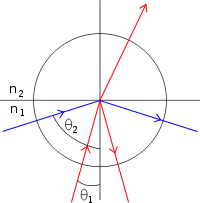The larger the angle to the normal, the smaller is the fraction of light transmitted, until the angle when total internal reflection (blue line) occurs. (The color of the rays is to help distinguish the rays, and is not meant to indicate any color dependence.)

Total internal reflection is an optical phenomenon that happens when a ray of light strikes a medium boundary at an angle larger than a particular critical angle with respect to the normal to the surface. If the refractive index is lower on the other side of the boundary and the incident angle is greater than the critical angle, no light can pass through and all of the light is reflected. The critical angle is the angle of incidence above which the total internal reflection occurs.

When light crosses a boundary between materials with different refractive indices, the light beam will be partially refracted at the boundary surface, and partially reflected. However, if the angle of incidence is greater (i.e. the ray is closer to being parallel to the boundary) than the critical angle – the angle of incidence at which light is refracted such that it travels along the boundary – then the light will stop crossing the boundary altogether and instead be totally reflected back internally. This can only occur where light travels from a medium with a higher [n1=higher refractive index] to one with a lower refractive index [n2=lower refractive index]. For example, it will occur when passing from glass to air, but not when passing from air to glass.

## Optical description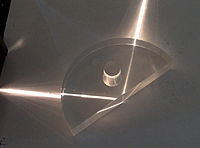Total internal reflection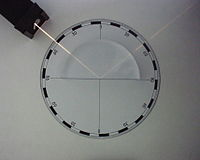Total internal reflection on plexi–air surface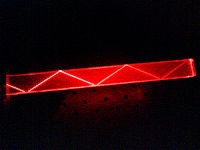Total internal reflection in a block of PMMA

Total internal reflections can be demonstrated using a semi-circular glass block. A "ray box" shines a narrow beam of light (a "ray") onto the glass. The semi-circular shape ensures that a ray pointing towards the centre of the flat face will hit the curved surface at a right angle; this will prevent refraction at the air/glass boundary of the curved surface. At the glass/air boundary of the flat surface, what happens will depend on the angle. Where θc is the critical angle measurement which is caused by the sun or a light source (measured normal to the surface):

• If θ < θc, the ray will split. Some of the ray will reflect off the boundary, and some will refract as it passes through.
• If θ > θc, the entire ray reflects from the boundary. None passes through. This is called total internal reflection.

This physical property makes optical fibers useful and prismatic binoculars possible. It is also what gives diamonds their distinctive sparkle, as diamond has an extremely high refractive index.

## Critical angleIllustration of Snell's law, nisin θi = ntsin θt.

The critical angle is the angle of incidence above which total internal reflection occurs. The angle of incidence is measured with respect to the normal at the refractive boundary (see diagram illustrating Snell's law). Consider a light ray passing from glass into air. The light emanating from the interface is bent towards the glass. When the incident angle is increased sufficiently, the transmitted angle (in air) reaches 90 degrees. It is at this point no light is transmitted into air. The critical angle θc is given by Snell's law,$n_i\sin\theta_i = n_t\sin\theta_t \quad$.

Rearranging Snell's Law, we get incidence$\sin \theta_i = \frac{n_2}{n_1} \sin \theta_t$.

To find the critical angle, we find the value for θi when θt = 90°. The resulting value of θi is equal to the critical angle θc.

Now, we can solve for θi, and we get the equation for the critical angle:$\theta_c = \theta_i = \arcsin \left( \frac{n_2}{n_1} \right),$

If the incident ray is precisely at the critical angle, the refracted ray is tangent to the boundary at the point of incidence. If for example, visible light were traveling through acrylic glass (with an index of refraction of 1.50) into air (with an index of refraction of 1.00). The calculation would give the critical angle for light from acrylic into air, which is$\theta _{c}=\arcsin \left( \frac{1.00}{1.50} \right)=41.8{}^\circ$.

Light incident on the border with an angle less than 41.8° would be partially transmitted, while light incident on the border at larger angles with respect to normal would be totally internally reflected.

If the fraction n2 / n1 is greater than 1, then arcsine is not defined—meaning that total internal reflection does not occur even at very shallow or grazing incident angles.

So the critical angle is only defined when n2 / n1 is less than 1.

## Derivation of evanescent wave

An important side effect of total internal reflection is the propagation of an evanescent wave across the boundary surface. Essentially, even though the entire incident wave is reflected back into the originating medium, there is some penetration into the second medium at the boundary. The evanescent wave appears to travel along the boundary between the two materials, leading to the Goos-Hänchen shift.

If a plane wave, confined to the xz plane, is incident on a dielectric with an angle θI and wavevector$\mathbf{k_I}$ then a transmitted ray will be created with a corresponding angle of transmittance as shown in Fig. 1. The transmitted wavevector is given by:$\mathbf{k_T}=k_T\sin(\theta_T)\hat{x}+k_T\cos(\theta_T)\hat{z}$

If n1 > n2, then sin(θT) > 1 since in the relation$\sin(\theta_T)=\frac{n_1}{n_2}\sin(\theta_I)$ obtained from Snell's law,$\frac{n_1}{n_2}\sin(\theta_I)$ is greater than one.

As a result of this cos(θT) becomes complex:$\cos(\theta_T)=\sqrt{1-\sin^2(\theta_T)}=i\sqrt{\sin^2(\theta_T)-1}$

The electric field of the transmitted plane wave is given by$\mathbf{E_T}=\mathbf{E_0}e^{i(\mathbf{k_T}\cdot\mathbf{r}-\omega t)}$ and so evaluating this further one obtains:$\mathbf{E_T}=\mathbf{E_0}e^{i(\mathbf{k_T}\cdot\mathbf{r}-\omega t)}=\mathbf{E_0}e^{i(xk_T\sin(\theta_T)+zk_T\cos(\theta_T)-\omega t)}$

and$\mathbf{E_T}=\mathbf{E_0}e^{i(xk_T\sin(\theta_T)+zk_Ti\sqrt{\sin^2(\theta_T)-1}-\omega t)}$.

Using the fact that$k_T=\frac{\omega n_2}{c}$ and Snell's law, one finally obtains$\mathbf{E_T}=\mathbf{E_0}e^{-\kappa z}e^{i(kx-\omega t)}$

where$\kappa=\frac{\omega}{c}\sqrt{(n_1\sin(\theta_I))^2-n^2_2}$ and$k=\frac{\omega n_1}{c}\sin(\theta_I)$.

This wave in the optically less dense medium is known as the evanescent wave. Its characterized by its propagation in the x direction and its exponential attenuation in the z direction. Although there is a field in the second medium, it can be shown that no energy flows across the boundary. The component of Poynting vector in the direction normal to the boundary is finite, but its time average vanishes. Whereas the other two components of Poynting vector (here x-component only), and their time averaged values are in general found to be finite.

## Superluminal energy transport

According to a paper published in April 2011 in Optics Communications, the velocity of transfer energy of the transmitted electromagnetic field in the optically thinner medium can exceed the light speed c of vacuum. However this violates a variety of physical laws.

## Frustrated total internal reflection

Under "ordinary conditions" it is true that the creation of an evanescent wave does not affect the conservation of energy, i.e. the evanescent wave transmits zero net energy. However, if a third medium with a higher refractive index than the low-index second medium is placed within less than several wavelengths distance from the interface between the first medium and the second medium, the evanescent wave will be different from the one under "ordinary conditions" and it will pass energy across the second into the third medium. (See evanescent wave coupling.) This process is called "frustrated" total internal reflection (FTIR) and is very similar to quantum tunneling. The quantum tunneling model is mathematically analogous if one thinks of the electromagnetic field as being the wave function of the photon. The low index medium can be thought of as a potential barrier through which photons can tunnel.

The transmission coefficient for FTIR is highly sensitive to the spacing between the high index media (the function is approximately exponential until the gap is almost closed), so this effect has often been used to modulate optical transmission and reflection with a large dynamic range. An example application of this principle is the multi-touch sensing technology for displays as developed at the New York University’s Media Research Lab.

## Phase shift upon total internal reflection

A lesser-known aspect of total internal reflection is that the reflected light has an angle dependent phase shift between the reflected and incident light. Mathematically this means that the Fresnel reflection coefficient becomes a complex rather than a real number. This phase shift is polarization dependent and grows as the incidence angle deviates further from the critical angle toward grazing incidence.

The polarization dependent phase shift is long known and was used by Fresnel to design the Fresnel rhomb which allows to transform circular polarization to linear polarization and vice versa for a wide range of wavelengths (colors), in contrast to the quarter wave plate. The polarization dependent phase shift is also the reason why TE and TM guided modes have different dispersion relations.

## Applications

• Optical fibers, which are used in endoscopes and telecommunications.
• Rain sensors to control automatic windscreen/windshield wipers.
• Another interesting application of total internal reflection is the spatial filtering of light.
• Prismatic binoculars use the principle of total internal reflections to get a very clear image
• Some multi-touch screens use frustrated total internal reflection in combination with a camera and appropriate software to pick up multiple targets.
• Gonioscopy to view the anatomical angle formed between the eye's cornea and iris.
• Gait analysis instrument, CatWalk, uses frustrated total internal reflection in combination with a high speed camera to capture and analyze footprints of laboratory rodents.
• Fingerprinting devices, which use frustrated total internal reflection in order to record an image of a person's fingerprint without the use of ink.
• Flashlights lenses.

## Examples in everyday life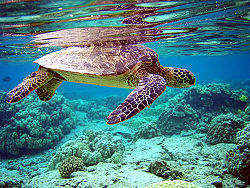Total internal reflection of the green turtle can be seen at the air-water boundary.

Total internal reflection can be observed while swimming, if one opens one's eyes just under the water's surface. If the water is calm, its surface appears mirror-like.

One can demonstrate total internal reflection by filling a sink or bath with water, taking a glass tumbler, and placing it upside-down over the plug hole (with the tumbler completely filled with water). While water remains both in the upturned tumbler and in the sink surrounding it, the plug hole and plug are visible since the angle of refraction between glass and water is not greater than the critical angle. If the drain is opened and the tumbler is kept in position over the hole, the water in the tumbler drains out leaving the glass filled with air, and this then acts as the plug. Viewing this from above, the tumbler now appears mirrored because light reflects off the air/glass interface.

Another very common example of total internal reflection is a critically cut diamond. This is what gives it maximum sparkle.

Wikimedia Foundation. 2010.

Нужна курсовая?

### Look at other dictionaries:

• total internal reflection — Optics. See total reflection. * * * Complete reflection of a ray of light in a medium such as water or glass, from the surrounding surfaces back into the medium. It occurs when the angle of incidence is greater than a certain limiting angle,… …   Universalium

• total internal reflection — Optics. See total reflection. * * * total internal reflection noun (physics) The complete reflection of a light ray at the boundary of a medium with a lower refractive index • • • Main Entry: ↑total …   Useful english dictionary

• total internal reflection — visiškasis vidaus atspindys statusas T sritis fizika atitikmenys: angl. total internal reflection vok. innere Totalreflexion, f rus. полное внутреннее отражение, n pranc. réflexion intégrée interne, f; réflexion totale interne, f …   Fizikos terminų žodynas

• Total internal reflection fluorescence microscope — (TIRFM) diagram 1. Specimen 2. Evanescent wave range 3. Cover slip 4. Immersion oil 5. Objective 6. Emission beam (signal) 7. Excitation beam ] 1. Objective 2. Emission beam (signal) 3. Immersion oil 4. Cover slip 5. Specimen 6. Evanescent wave… …   Wikipedia

• total internal reflection — noun the total reflection of a light ray which occurs when light from one medium strikes the surface of a medium of lower refractive index or optical density in excess of the critical angle …   Australian-English dictionary

• Total Internal Reflection —   The trapping of light by refraction and reflection at critical angles inside a semiconductor device so that it cannot escape the device and must be eventually absorbed by the semiconductor …   Energy terms

• total internal reflection —   The trapping of light by refraction and reflection at critical angles inside a semiconductor device so that it cannot escape the device and must eventually be absorbed by the semiconductor.   Solar Electric Glossary …   Energy terms

• total internal reflection — noun Physics the complete reflection of light (without refraction), occurring at an interface with a less dense medium when the angle of incidence exceeds the critical angle …   English new terms dictionary

• Total external reflection — is an optical phenomenon where electromagnetic radiation (e.g. visible light) can, at certain angles, be totally reflected from an interface between two media of different indices of refraction (see Snell s law). Total internal reflection occurs… …   Wikipedia

• Reflection (physics) — Reflection is the change in direction of a wave front at an between two different media so that the wave front returns into the medium from which it originated. Common examples include the reflection of light, sound and water waves. Law of… …   Wikipedia